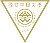# 最新消息

##演講通知-Prof. Victor Hugo Lachos Davila 2023/09/27 (三)、09/28 (四) 11:10-12:00 AM

### 2023年09月06日

Abstract：

The EM algorithm is a popular tool for maximum likelihood estimation but has not been used much for high-dimensional regularization problems in linear mixed-effects models. In this paper, we introduce the EMLMLasso algorithm, which combines the EM algorithm and the popular and efficient R package glmnet for Lasso variable selection of fixed effects in linear mixed-effects models. We compare the performance of our proposed EMLMLasso algorithm with the one implemented in the well-known R package glmmLasso through the analyses of both simulated and real-world applications. The simulations and applications demonstrated good properties, such as consistency, and the effectiveness of the proposed variable selection procedure, for both pn. Moreover, in all evaluated scenarios, the EMLMLasso algorithm outperformed glmmLasso. The proposed method is quite general and can be easily extended for ridge and elastic net penalties in linear mixed-effects models.

Abstract：

The Heckman selection model is perhaps the most popular econometric model in the analysis of data with sample selection. The analyses of this model are based on the normality assumption for the error terms, however, in some applications, the distribution of the error term departs significantly from normality, for instance, in the presence of heavy tails and/or atypical observation.  In this paper, we explore the Heckman selection-t model where the random errors follow a bivariate Student's-t distribution.  We develop an analytically tractable and efficient EM-type algorithm for iteratively computing maximum likelihood estimates of the parameters, with standard errors as a by-product. The algorithm has closed-form expressions at the E-step, that rely on formulas for the mean and variance of the truncated Student's-t distributions. Simulation studies show the vulnerability of the Heckman selection-normal model, as well as the robustness aspects of the Heckman selection-t model. Two real examples are analyzed, illustrating the usefulness of the proposed methods. The proposed algorithms and methods are implemented in the new R package HeckmanEM.